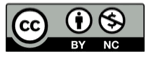# Python 展开多层嵌套的列表

2018-04-24## 背景

``````[1, 'a', ['b', ['c'], [ ], [3, 4]]]
``````

``````1, a, b, c, 3, 4
``````

## 未知嵌套层数

``````def 展开（任意序列）
``````

``````def 展开（任意序列）:
对于 任意序列 里的 每一项:
如果 仍然是序列:
给出（yield）展开（这个序列） 里的每一项
否则：
给出（yield）这一项
``````

``````def flatten(sequence):
for item in sequence:
if type(item) is list:
for subitem in flatten(item):
yield subitem
else:
yield item
a = [1, 'a', ['b', ['c'], [ ], [3, 4]]]
for x in flatten(a):
print x,
``````

## 用列表解析实现

``````flatten = lambda x: [subitem for item in x for subitem in flatten(item)] \
if type(x) is list else [x]
a = [1, 'a', ['b', ['c'], [ ], [3, 4]]]
for x in flatten(a):
print x,
``````

``````1 a b c 3 4
``````

## 用 reduce 实现

from functools import reduce

``````reduce(lambda x,y:x+y,[[1,2],[3,4]])
``````

``````[1, 2, 3, 4]
``````

## 精彩评论